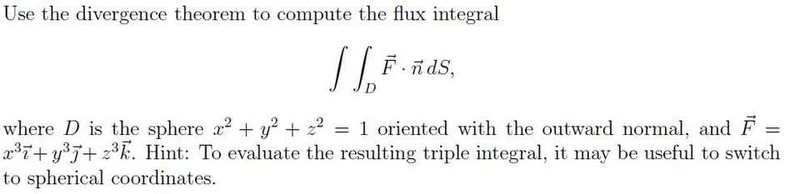# Use Divergence Theorem to Compute the Flux Integral Just a work check!Alright so I found div F=3x2+3y2+3z2

The integral then becomes the triple integral of the divergence of F times the derivative of the volume.

Changing into spherical coordinates, the integral becomes $$3\int_{0}^{2\pi}\int_{0}^{\pi}\int_{0}^{1}p^{4}sin{\phi}dpd{\phi}d{\theta}$$ which ends up equaling $$2.4{\pi}$$.

Is this correct?

Last edited: